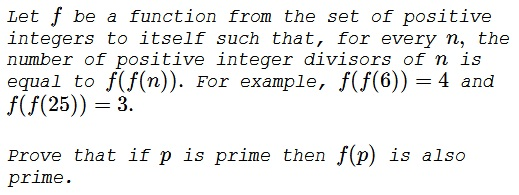# Problem 2 from the 2017 Canada MO

### Problem### Solution 1

$p$ is a prime iff $f(f(p))=2.$ In particular, $f(f(2))=2.$ To solve the problem, suffice it to establish that $f(2)=2.$ Indeed, taking $p$ prime, $f(f(p))=2,$ from which $f(f(f(p)))=f(2)=2,$ meaning that $f(p)$ is also prime.

Note, for the record, that $f(f(n))=1,$ iff $n=1.$ So assume that, $f(2)\ne 2.$ If $f(2)=n\ne 2$ then $2=f(f(2))=f(n)$ and, subsequently, $n=f(2)=f(f(n)).$ But, for any $k,$ $f(f(k))\le k,$ because all the factors of a number are less than, or equal to, that number. More than that, for $k\gt 2,$ $\gcd(k,k-1)=1,$ so that $k\gt 2\Rightarrow\, k\gt f(f(k)).$ Thus, $n=f(2)=f(f(n))$ implies $n\le 2.$

Assume $f(2)=1.$ From $1=f(2)$ it follows that $f(1)=f(f(2))=2.$ Also, for a prime $p,$ $f(f(f(p)))=f(2)=1,$ implying $f(p)=1,$ for any prime $p.$

Finally, since $3$ is a prime and $f(f(25))=3,$ we see that $f(f(f(25)))=f(3)=1,$ so that $f(25)=1.$ But then $3=f(f(25))=f(1)=2.$ A contradiction. Thus, $f(2)=2.$

### Solution 2

Let $d(n) = f(f(n))$ denote the number of divisors of $n$ and observe that

$f(d(n)) = f(f(f(n))) = d(f(n)),$

for all $n.$ Also note that because all divisors of $n$ are distinct positive integers between $1$ and $n,$ including $1$ and $n,$ and excluding $n - 1$ if $n \gt 2,$ it follows that $2 = d(n) \lt n$ for all $n \gt 2.$ Furthermore $d(1) = 1$ and $d(2) = 2.$

We first will show that $f(2) = 2.$ Let $m = f(2)$ and note that

$2 = d(2) = f(f(2)) = f(m).$

If $m = 2,$ then let $m_0$ be the smallest positive integer satisfying that $m_0 = 2$ and $f(m_0) = 2.$ It follows that

$f(d(m_0)) = d(f(m_0)) = d(2) = 2.$

By the minimality of $m_0,$ it follows that $d(m_0) = m_0,$ which implies that $m_0 = 2.$ Therefore if $m = 2,$ it follows that $f(2) = 2.$ It suffices to examine the case in which $f(2) = m = 1.$ If $m = 1,$ then $f(1) = f(f(2)) = 2$ and, furthermore, each prime $p$ satisfies that $d(f(p)) = f(d(p)) = f(2) = 1$ which implies that $f(p) = 1.$ Therefore $d(f(p 2)) = f(d(p^2)) = f(3) = 1$ which implies that $f(p^2) = 1$ for any prime $p.$ This implies that $3 = d(p^2) = f(f(p^2)) = f(1) = 2,$ which is a contradiction.

Therefore $m\ne 1$ and $f(2) = 2.$ It now follows that if $p$ is prime then $2 = f(2) = f(d(p)) = d(f(p))$ which implies that $f(p)$ is prime.

### Acknowledgment

This is problem 2 from the 2017 Canada Mathematical Olympiad. The complete list of the olympiad problems has been posted at the Crux Mathematicorum facebook page. Solution 2 is the official one.Copyright © 1996-2018 Alexander Bogomolny

68545573